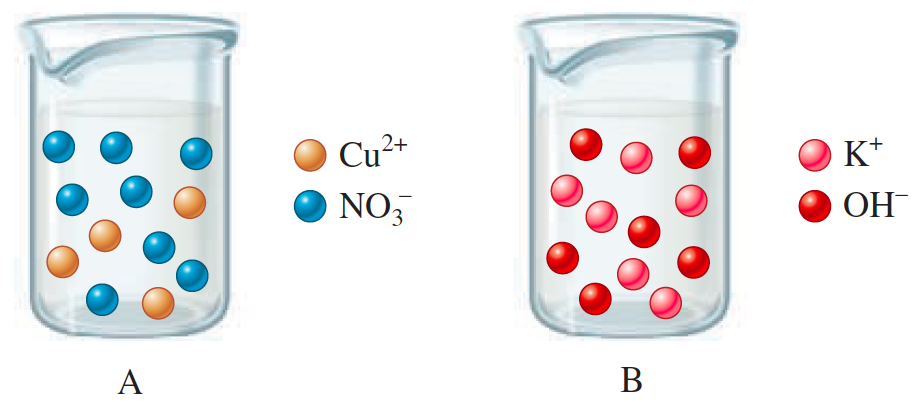# Problem: The drawings below represent aqueous solutions. Solution A is 2.00 L of a 2.00-M aqueous solution of copper(II) nitrate. Solution B is 2.00 L of a 3.00-M aqueous solution of potassium hydroxide.Determine the concentrations (in M) of all ions left in solution after mixing and the mass of solid formed.

###### FREE Expert Solution

Step 1

Reactant

Co(NO3)2(aq) Co2+ (aq) + 2 NO3- (aq)

KOH (aq) K+ (aq) + OH- (aq)

Reaction: double displacement

Product

Co2+ (aq) + 2 OH- (aq) Co(OH)2 (s)

K+ (aq) + NO3- (aq) KNO3 (aq)

Balance equation for the reaction:

Co(NO3)2(aq) + 2 KOH (aq) → Co(OH)2 (s) + 2 KNO3 (aq)

Step 2

= 4.00 mol Co(OH)2

= 6.00 mol KOH

Step 3

from Co(NO3)2(aq)

= 4.00 mol Co(OH)2

from KOH

= 3.00 mol Co(OH)2

limiting reactant → KOH

85% (17 ratings)###### Problem Details

The drawings below represent aqueous solutions. Solution A is 2.00 L of a 2.00-M aqueous solution of copper(II) nitrate. Solution B is 2.00 L of a 3.00-M aqueous solution of potassium hydroxide.Determine the concentrations (in M) of all ions left in solution after mixing and the mass of solid formed.